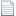# 公平性指派问题及均值逼近求解算法 ： 三次分配问题（NP-hard）的四次多项式时间精确求解

创新点2：提出了一类具有广泛应用性的指数分配问题

(上海理工大学 管理学院，上海 200093)

Fair Assignment Problem and its Average Approximation Algorithm

He Shengxue

(Business School, University of Shanghai for Science and Technology, Shanghai 200093, China)

AbstractTo balance the assigned working loads among agents, this paper formulated a fair assignment optimization model and proposed a numerical method for obtaining the global optimal solution. By defining the sum of squares of differences between assigned working loads of agents and the average load as the index of measuring the fairness of working load, this paper constructed the fair assignment model combining the constraints of the classic assignment problem. This study classified the problem according to whether the working load matrix could be decomposed into two special matrices and pointed out that a fair assignment problem is in general a type of cubic assignment problem. By regularly going through the feasible range of working load, this paper transformed the solution process into a one-dimensional search process that needs to solve a series of classic linear assignment problems. This study proved theoretically the soundness of the average approximation algorithm and pointed out the quartic polynomial time complexity concerning the scale of the problem. By comparing with the results of commercial optimization software-Lingo, the comparison verified that the new method obtains the global optimal solution and needs very low computational time.

Key words: integer programming; NP-hard problem; approximation algorithm; combinational optimization; assignment problem公平性指派问题及均值逼近求解算法_何胜学.pdf

https://blog.sciencenet.cn/blog-3367056-1345914.html

## 全部精选博文导读

GMT+8, 2022-8-18 13:56